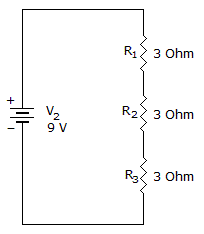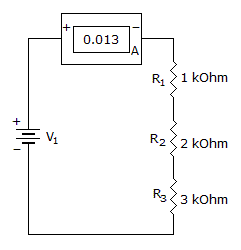# Electronics - Series Circuits

Exercise : Series Circuits - General Questions
1.
What is the current flow through R1, R2, and R3?1A, 1A, 1A
1A, 2A, 3A
3A, 3A, 3A
3A, 2A, 1A
Explanation:

V = IR

V = I(R1 + R2 + R3)

I = V/(R1 + R2 + R3)

= 9/(3 + 3 + 3)

= 9/9

= 1 Amp.

2.
One of the most common applications of a potentiometer is as an adjustable voltage divider, also known as
voltage control
current control
volume control
divider control
Explanation:
No answer description is available. Let's discuss.

3.
If the resistance total in a series circuit doubles, current will:
be the same
be doubled
reduce source voltage
be halved
Explanation:
No answer description is available. Let's discuss.

4.
Power is defined as:
the rate at which work is done
work
the conversion of energy
joules
Explanation:
No answer description is available. Let's discuss.

5.
What is the dc source voltage?78 V
39 V
13 V
0 V